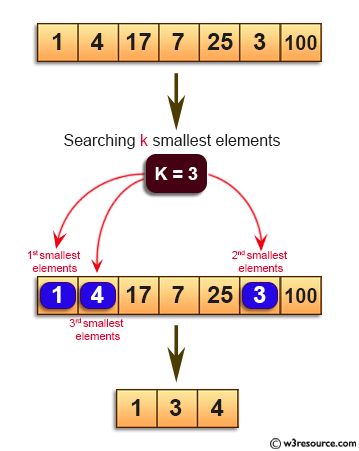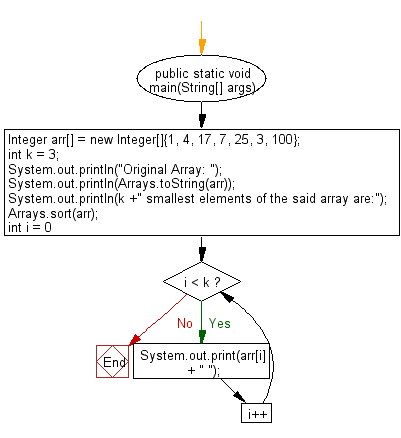﻿ Java programming exercises: Find the k smallest elements in a specified array - w3resource# Java Exercises: Find the k smallest elements in a specified array

## Java Basic: Exercise-160 with Solution

Write a Java program to find the k smallest elements in a given array. Elements in the array can be in any order.

Pictorial Presentation:Sample Solution:

Java Code:

``````import java.util.*;
public class Solution {

public static void main(String[] args)
{
Integer arr[] = new Integer[]{1, 4, 17, 7, 25, 3, 100};
int k = 3;
System.out.println("Original Array: ");
System.out.println(Arrays.toString(arr));
System.out.println(k + " smallest elements of the said array are:");
Arrays.sort(arr);
for (int i = 0; i < k; i++)
System.out.print(arr[i] + " ");
}
}
```
```

Sample Output:

```Original Array:
[1, 4, 17, 7, 25, 3, 100]
3 smallest elements of the said array are:
1 3 4
```

Flowchart:Java Code Editor:

What is the difficulty level of this exercise?

﻿

## Java: Tips of the Day

Parsing dates:

```import java.io.*;
import java.util.*;
import java.text.*;

String s = "2001/09/23 14:39";

SimpleDateFormat formatter = new SimpleDateFormat ("yyyy/MM/dd H:mm");
Date d = formatter.parse(s, new ParsePosition(0));
```